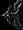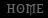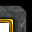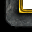Tutorial By Effane

Effane's Save/Load Code tutorial for Intermediate Trigger Makers.

I.Part 1
2. How do the Alpha Numbers work
3. Setting up an initialization trigger
Part II.
4. Setting up a Save Code Trigger
Part III.
5. Setting up a Load Code Trigger
Part IV.
7. Sample Code FAQ

Part I

Any WC3 map that requires players to play for very long time periods will most likely need a save code. Even some short game maps like AOS and TDs use save/load codes for transferring player stats from one game to the next.
Now these Save/Load Codes can be very powerful, and very complicated. I hope by the end of this tutorial you have a solid grasp of at least the basic parts of the Save/Load Codes.

These codes are usually managed by systems of triggers. Most codes use either 2 or 3 triggers. I personally perfer to use 3 triggers to break down complexity and to save on process power. This does come at the cost of memory, but not that much.

The three triggers I use are pretty simple categories: Initialization, Save, and Load.
Initialization Trigger, this sets up all the variables, loads the basic Alphanumbers and does all the ground work so players can have everything they need for the next two triggers.
Save, this is obviously the trigger that collects the information and then converts it to a text file.
Load, this is the trigger that takes the Save Code and converts it back into information that can be used to load all the information you had the save code save.

The whole system is based off a simple idea. Change the size of the counting system to a higher number, convert the data to match that and give it to the player to write down. Then reverse the process once the player gives you the information back the next time. The basic tool for all of this is the AlphaNumber system.

2. How do the Alpha Numbers work

AlphaNumbers. These are the whole core of the system for Save/Load Codes. This is why its so important to know what they are and how they do the work for us.
Most people know about two types of number systems already that are around computers. The Binary and the Decimal system. There are two numbers in Binary 0 and 1, while Decimal of course has 10 numbers (0123456789). You could basically veiw these as AlphaNumbers of system 2 and 10.
Now, obviously 10 digit system is alot more compact than the 2 digit system. The number 32 in Decimal is only 2 spaces used, while in binary its 100000 or six digits used. Well if you created a number system greater than 32 number, it would give you a single digit to hold that number.
For almost all of this tutorial I will be using a simple 36 character Alphanumber system as follows: 0123456789ABCDEFGHIJKLMNOPQRSTUVWXYZ. With 36 numbers alone, we can compress alot of larger numbers into much smaller digits, saving alot of space.

How do you read numbers like this? Well the number system will go from 0-35. You have to multiply and divide to move from a lower digit to a higher one. The decimal number 36 is actually 10 is this number system. Since it went over the max char of 35, it adds one place further out to the left. While 1296 is 100. Now when you are counting from 34-37 for example it will go like this.

34 = 0Y
35 = 0Z
36 = 10
37 = 11

Now how to convert from Decimal to AlphaNumbers and back.

You need to be able to move back and forth between these two systems. Now basic algebra and loops will be our friend here.

First you have to get your Decimal number. We will work with 123456. And in our 36 character number system.

Now when converting number systems, if you go from little to big, you divide. From bigger to little, you multiply. Simple rule of thumb. For saving from now on, we will always divide (Why would you use less than 10 digits for saving) and for loading we will multiply, keep this in mind.

Since 123456 is Base 10 and we are using 36 character system here we go with dividing. Now keep in mind integer math in WC3 triggers is limited. And we need variables to work from anyways so we will do it all with just four things, + - x /.

-------- Slot1=36 --------
-------- Slot2=1296 --------
-------- Slot3=46656 --------
-------- Slot4=1679616 --------
-------- Slot5=60466176 --------

Now we have to start from the smallest number we can fit the number in the system. Lets say this is a measurement of Player Gold, which by default maxes at 1000000. So we are using a 4 character number here (36x36x36x36=1.6 million).

First we divide by the max character slot minus 1. So we divide by 36x36x36 or 36^3 = 46656.

123456 / 46656 = 2.26... (Now we are going to use just integers for all of this, so everything after the decimal point will get dropped, no rounding at all). => 2.
So we know our first character is number 2 in the first string slot. Which in our number system is 2 actually.

Now we need to get our remainder. You will see later how we achieve that in code, but for now its just = 30144.

Now we go down one power of 36 to 36^2 or 1296. Once again we divide our remainder by 1296 and get the results. 30144 / 1296 = 23.59... or 23. Which converts to N.

Remainder and again we divide by 36^1. 336 / 36 = 9.33... or just 9. Which is equal to 9.

Final run is 36^0 or 1 (Anything to the power of zero is always just 1). 12 / 1 = 12 or C, you should never have a remainder with conversions like this. If you do, then OOOPs....

Now the new number is 2N9C. Not bad for making it smaller.

Now we need to make it go back to a Decimal number. That of course takes Multiplying like I said before. We will start from the left and move right just like above.

To do this we will take the power of 36 to the slot of the Alphanumber, then multiply it against the characters number position and add it all together when you have all the slots done.

2### => 2 x 36^3 => 2 X 46656 = 93312
#N## => 23 x 36^2 = 23 X 1296 = 29808
##9# => 9 X 36^1 = 9 X 36 = 324
###C => 12 X 36^0 = 12 X 1 = 12
-----------------------------------------
123456

Now that we know how to use the number system, we need to start building it. This will require a trigger system.

3. Setting up an initialization trigger

This is the groundwork of our entire trigger. As mentioned before, some people like to include this in their save and load code, but I think its much more efficient to save it in its own trigger and it raises the ability to manage the triggers size by alot, these codes cant get very huge afterall, such as my 57 save/load code in my ORPG consisting of 21 objects.

Here is the demo Intialization trigger included in the code in its entirety.
I will break it down into pieces after this Code Block.

Trigger setup time can actually be on initialization, but I dont personally trust WC3 to load things properly in that spot, and honestly you can load the trigger after a .10 second while your doing something else like broadcasting info, showing a movie, whatever that will keep the player busy while its loading.

-------- Need to load your number system in here, right now its 36 chars. -------- -------- Slot1=36 -------- -------- Slot2=1296 -------- -------- Slot2=1296 -------- -------- Slot3=46656 -------- -------- Slot4=1679616 -------- -------- Slot5=60466176 -------- -------- I like making 0 the actual 0 for the unencrypted system. makes it easier to track stuff later. -------- Set SaveLoadCharacterSet = 0123456789ABCDEFGHIJKLMNOPQRSTUVWXYZ Set SaveLoadMaxCharacters = 36

This first block of code is basically notes, it helps alot to use a calculator and make your numbers ahead of time so you know how many slots you need.
The SaveLoadCharacterSet variable is a string that holds your number system. Make sure you avoid things like Spaces, and in this systems case, Dashes and Lower Case Chars. Also keep in mind your players need to type these codes in. Not everyone is a typing master.

-------- Need to load your encryption system in here, right now its 5 sets. Load code will get broken if you use a '-' in the Number system -------- Set SaveLoadEncryptionSet = ABCDEFGHIJKLMNOPQRSTUVWXYZ0123456789 Set SaveLoadEncryptionSet = GHIJKLMNOPQRSTUVWXYZ0123456789ABCDEF Set SaveLoadEncryptionSet = ABCDEFPQRSTUVWXYZ012345GHIJKLMNO6789 Set SaveLoadEncryptionSet = ASTUVWXYBCJKLMNOPQRZ01234DFEGHI56789 Set SaveLoadEncryptionSet = HIJKLABCDEVWXYZ0123FGM4567NOPQRSTU89 Set SaveLoadMaxEncryptionSets = 5

The above code creates an encryption system. While this is crucial for game purposes, right now its nice to know it just exist. I will cover this more later.

-------- Now time to populate the Number system. this loop will put the characters into an array which acts like a counting system from 0 till MaxChars -------- For each (Integer A) from 1 to SaveLoadMaxCharacters, do (Actions) Loop - Actions Set SaveLoadCharacterNumbers[((Integer A) - 1)] = (Substring(SaveLoadCharacterSet, (Integer A), (Integer A)))

Here is the code that builds your Number system. Since we cant make a computer count from 0-Z we have to instead give it a way to count using something it already knows, the Decimal system. We do this by assigning each letter in our CharacterSet to an index in an Array (SaveLoadCharacterNumbers[]). As you may have noticed, the array goes from 0-35, not 1-36. This is so you can use the 0, without it our number system wouldnt be the same.

This here is the base level setup for the items to be stored. Notice Items and Heroes are in an array ahead of time. This is so they can converted to decimal equivalancies which will make it much easier to handle, and also shrinks the information size down alot.
At the end of each section you will notice a Slots variable. This is an integer that holds how many chars each variable uses. You can change this to modify the size of information that will be stored. Refer to the Slots# comments at the very beginning for idea how much each variable will enable you to hold.

This code was intended for a loop action in the save/load code, but I never implemented it. So its really not used. BUT this code does give you a chance to map out where you plan to have your characters in order at.

-------- This variable stops players from loading again after they load the first time. -------- For each (Integer A) from 1 to 4, do (Actions) Loop - Actions Set SaveLoadHasLoaded[(Integer A)] = False

This code isnt really necessary, but its very good to have safety code like this. If the player typed in -load twice, they could be in trouble if you didnt have something to track them. This action is just a redundant check to make sure they can load properly.

Part 2
Part 3
Part 4